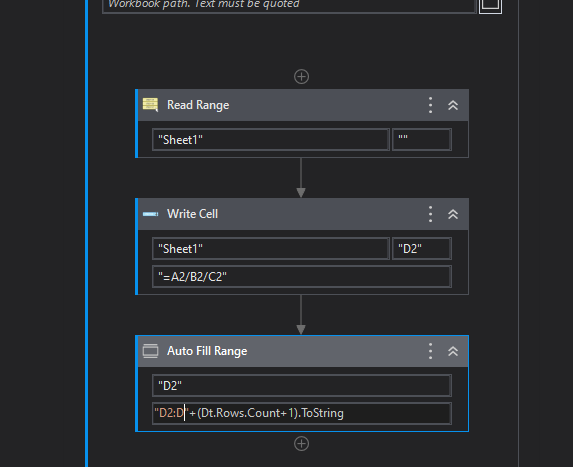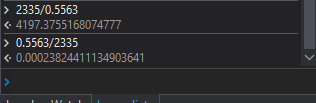# Uipath Excel cell divide

Hello…
I have Two variable and that name is variable1 and Variable2.

And I need to this two variable divide answer write down in new variable3 cell.

So, can you help me like which expression i used.

HI @rAE_rAS

Use Read range activity → Store it as `DtRead`

Use For each row in Data Table activity

Use Assign activity

``````Currentrow("Col3") = Cint(Currentrow("Column1").Tostring) / Cint(Currentrow("Column2").Tostring)
``````

Redards
Gokul

HI @rAE_rAS

Try this way

Let say the values are in the column A and B and answer should be in C

Regards
Sudharsan

But i have mentioned " " is not possible because my value is variable

Not possible because i have three varible

Share the sample input @rAE_rAS

I’m Quite confused @rAE_rAS

You have three variables? Can you edit your question?

You can try like thisRegards
Sudharsan

Use “D”+int_raw.ToString

What is Variable 1 and Variable 2? Unclear information @rAE_rAS

Varible1 means somevalue and and varible2 name means some value

And? @rAE_rAS

What do you mean by Some Value @rAE_rAS

Give us more details to solve your issue

Variable1 means like 2335, and Varible2 means like 0.5563

Can you share a sample input file ? @rAE_rAS

Regards
Sudharsan

You can try like this `Variable1 /Variable2` @rAE_rAS